### Home > MC2 > Chapter 6 > Lesson 6.1.5 > Problem6-50

6-50.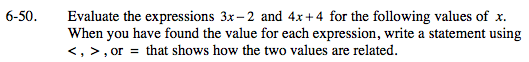Substitute the given values for x and decide whether one side is greater than, less than, or equal to the other side.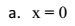3 ( 0 ) − 2 = 0 − 2 = −2

4 ( 0 ) + 4 = 0 + 4 = 4

−2 < 4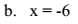3 ( −6 ) − 2 = −18 − 2 = −20

4 ( −6 ) + 4 = −24 + 4 = −20

−20 = −20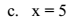3 ( 5 ) − 2 = ?

4 ( 5 ) + 4 = ?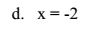Refer to parts (a), (b), and (c).